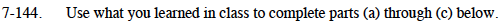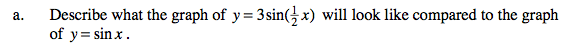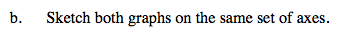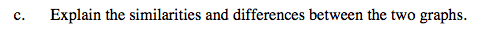Home > CCA2 > Chapter 7 > Lesson 7.2.3 > Problem7-144

7-144.
1. Use what you learned in class to complete parts (a) through (c) below. 7-144 HW eTool (Desmos). Homework Help ✎

1. Describe what the graph of y = 3sin(x) will look like compared to the graph of y = sin x.

2. Sketch both graphs on the same set of axes.

3. Explain the similarities and differences between the two graphs.Is there a horizontal or vertical shift? Does the amplitude or period change?

$\text{The graph of }y = 3\text{sin} \left(\frac{1}{2}x\right)\text{ has an amplitude of 3 and a period of } 4\pi.$Since there is no horizontal or vertical shift, both graphs start at (0,0).See parts (a) and (b).

Use the eTool below to help answer the parts of the problem.
Click the link at right for the full version of the eTool: CCA2 7-144 HW eTool